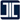『您的瀏覽器不支援JavaScript功能，若網頁功能無法正常使用時，請開啟瀏覽器JavaScript狀態』

# 工業技術研究院

:::

## 產業服務

### 技術名稱： 管路流量量測系統及其方法

#### Abstract

A tube flow rate measurement system is configured to measure a fluid flow rate of a fluid passing through a test segment of a tube, and the fluid flow rate measurements system comprises a first sensor set, a second sensor set, and a computing device. The first sensor set and the second sensor set are respectively configured to connect with two ends of the test segment, and the computing device is electrically connected to the first sensor set and the second sensor set. The computing device calculates a fluid velocity set according to a plurality of sensing signal pairs transmitted between the first sensor set and the second sensor set, and the computing device stores a plurality of classifications which is generated by executing a supervised learning many times and a fluid flow rate correction coefficient database. The computing device determines a flow field type of the fluid velocity set according to the classifications, and obtains a fluid flow rate correction coefficient corresponding to the flow field type of the fluid velocity set from the fluid flow rate correction coefficient database, and calculate the fluid flow rate according to the fluid flow rate correction coefficient and the fluid velocity set.

#### Technical Specification

A pipeline flow measurement system for measuring the flow of fluid in a section to be measured of a pipeline, the pipeline flow measurement system comprising: a first sensor group and a second sensor group , the first sensor group and the second sensor group are respectively assembled at different two ends of the to-be-measured segment; and a computing device is electrically connected to the first sensor group and the second sensor group a sensor group, and calculates a flow rate combination according to a plurality of sensing signal pairs transmitted between the first sensor group and the second sensor group; wherein the computing device A plurality of classification algorithms and a flow correction coefficient database generated by supervised learning, the computing device determines the flow field type to which the flow velocity combination belongs according to the classification algorithms, and the computing device obtains from the flow correction coefficient data The library obtains a flow rate correction coefficient corresponding to the type of the flow field, and the computing device calculates the flow rate according to the combination of the flow rate correction coefficient and the flow rate. A method for measuring the flow rate of a pipeline, for measuring the flow rate of a fluid in a section to be measured of a pipeline, comprising: using a computing device to read and transmit a first sensor group and a second sensor a plurality of pairs of sensing signals between the device groups; a flow velocity combination of the fluid is calculated by the computing device according to the pairs of sensing signals, wherein the computing device stores a plurality of classification algorithm and a flow correction coefficient database; use the classification algorithms to determine the type of flow field to which the flow velocity combination belongs; obtain a flow correction corresponding to the flow field type from the flow correction coefficient database with the flow field type coefficient; and calculating the flow rate according to the flow velocity combination and the flow rate correction coefficient by the computing device.

#### 聯絡資訊